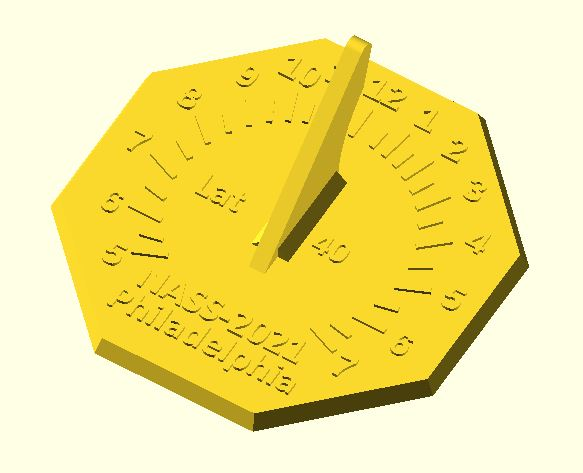Hits: 587

Here in the last in our series on making a sundial using OpenSCAD we need to finish the dial by draw the hour numbers. We know that the hour angle as measured at the foot of the gnomon is:
tan(theta) = sin(lat)*sin(HA)

where   HA is the hour angle from noon (15 deg for each hour)
lat is the latitude
theta is the hour line

we can rewrite this in an unambiguous way using the computer mathematical function atan2(x,y)

theta = atan2(sin(lat)*sin(HA), cos(HA))

But the gnomon is offset by some distance south of the dial center, an amount we'll call "goffset". (+ means that we've moved the gnomon to the north, - means we've moved the gnomon to the south). We want our numbers set at a distance of "crad" from the center.
In algebra class remember that quadratic equation that was hard to memorize and you thought you'd never use it again? Well here's your opportunity to put some nearly forgotten mathematics to use.

Trigonometry tells us that the distance from the gnomon to the hour number is different than the constant value of "crad". This distance "zo" is found using a version of the Pythagorean theorem of the squares of sides:

$$zo^2=crad^2 + goffset^2 - 2*crad*goffset*cos(theta)$$

The solution to zo is using the quadratic equation:

$$zo = \frac{bo - \sqrt{bo^2-4*ao*co}}{2*ao^2}$$

where
ao = 1
bo = -2*goffset*cos(theta)
co = goffset^2 - crad^2

The idea is to set up a loop for drawing the hour numbers. Let's say that we want to put down the numbers 5(am) to 7(pm). On a 24 hour scale, that's H = 5:19. Meanwhile that hour angle referenced to the 12-noon hour is HA = 15*(H-12) degrees.

Here's the idea of what our module might look like:module draw_hour_number(HA,H,gnomon_width) {
//text of one hour number
if (H>12) {
txt3 = str(H-12);
}else{
txt3 = str(H)
}

//classic hour line
theta = atan2(sin(latitude)*sin(HA),cos(HA));

if H {
wo = gnomon_width;
}else{
wo = -gnomon_width;
}

//we need to contend with the fact that the hour line extends from the gnomon foot.
//this is a distance goffset from the dial center. We need to use the quadratic
//equation to get the distance from the gnomon foot to a circular arc (chapter ring)
//of radius crad where our numbers will be placed...

push = 1.15;                                //tweak to move the number a bit further out on the dial face
ao     = 1;
bo     = -2*goffset*cos(theta);
zo     = push*abs((-bo - sqrt(bo*bo-4*co))/(2*ao));

//translate this to the dial x,y coordinate system
xo = zo*sin(theta) + wo/2;                   //remember that wo may be positive or negative
yo = zo*cos(theta) + goffset;                //goffset is a negative number since moved gnomon south of center

//print the number
translate([xo,yo,dial_thickness])            //raise the number to sit at the top of the dial face thickness
linear_extrude(height = ho)                  //ho is the thickness (height) of our number
text(txt3, size = 4.5, halign = "center",font = str("Angsana:style=Bold"), \$fn = 16);

}

The final OpenSCAD code to create this simple horizontal sundial is attached.  Download it and have fun.